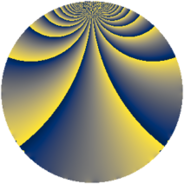# Properties

 Label 3800.1.bdLevel $3800$ Weight $1$ Character orbit 3800.bd Rep. character $\chi_{3800}(1451,\cdot)$ Character field $\Q(\zeta_{6})$ Dimension $14$ Newform subspaces $6$ Sturm bound $600$ Trace bound $11$

# Related objects

## Defining parameters

 Level: $$N$$ $$=$$ $$3800 = 2^{3} \cdot 5^{2} \cdot 19$$ Weight: $$k$$ $$=$$ $$1$$ Character orbit: $$[\chi]$$ $$=$$ 3800.bd (of order $$6$$ and degree $$2$$) Character conductor: $$\operatorname{cond}(\chi)$$ $$=$$ $$152$$ Character field: $$\Q(\zeta_{6})$$ Newform subspaces: $$6$$ Sturm bound: $$600$$ Trace bound: $$11$$

## Dimensions

The following table gives the dimensions of various subspaces of $$M_{1}(3800, [\chi])$$.

Total New Old
Modular forms 44 26 18
Cusp forms 20 14 6
Eisenstein series 24 12 12

The following table gives the dimensions of subspaces with specified projective image type.

$$D_n$$ $$A_4$$ $$S_4$$ $$A_5$$
Dimension 14 0 0 0

## Trace form

 $$14 q + q^{2} - q^{3} - 3 q^{4} - q^{6} - 2 q^{8} - 4 q^{9} + O(q^{10})$$ $$14 q + q^{2} - q^{3} - 3 q^{4} - q^{6} - 2 q^{8} - 4 q^{9} - 2 q^{11} + 2 q^{12} - 2 q^{14} - 7 q^{16} + 2 q^{17} + 3 q^{19} - q^{22} - q^{24} + 8 q^{26} - 2 q^{27} + q^{32} + q^{33} + 2 q^{34} - 8 q^{36} - 2 q^{38} + q^{41} + 2 q^{43} - 3 q^{44} - 4 q^{46} - q^{48} + 10 q^{49} + 4 q^{51} - 11 q^{54} - 4 q^{56} + 2 q^{57} + 9 q^{59} + 6 q^{64} + 7 q^{66} - q^{67} - 4 q^{68} - q^{73} - 2 q^{74} + 5 q^{76} - 9 q^{81} - q^{82} + 2 q^{83} - 10 q^{86} + 2 q^{88} + 4 q^{91} - 8 q^{94} + 2 q^{96} - q^{97} + q^{98} + 4 q^{99} + O(q^{100})$$

## Decomposition of $$S_{1}^{\mathrm{new}}(3800, [\chi])$$ into newform subspaces

Label Dim $A$ Field Image CM RM Traces $q$-expansion
$a_{2}$ $a_{3}$ $a_{5}$ $a_{7}$
3800.1.bd.a $2$ $1.896$ $$\Q(\sqrt{-3})$$ $D_{3}$ $$\Q(\sqrt{-2})$$ None $$-1$$ $$-2$$ $$0$$ $$0$$ $$q+\zeta_{6}^{2}q^{2}+\zeta_{6}^{2}q^{3}-\zeta_{6}q^{4}-2\zeta_{6}q^{6}+\cdots$$
3800.1.bd.b $2$ $1.896$ $$\Q(\sqrt{-3})$$ $D_{3}$ $$\Q(\sqrt{-2})$$ None $$-1$$ $$1$$ $$0$$ $$0$$ $$q+\zeta_{6}^{2}q^{2}-\zeta_{6}^{2}q^{3}-\zeta_{6}q^{4}+\zeta_{6}q^{6}+\cdots$$
3800.1.bd.c $2$ $1.896$ $$\Q(\sqrt{-3})$$ $D_{3}$ $$\Q(\sqrt{-2})$$ None $$1$$ $$-1$$ $$0$$ $$0$$ $$q-\zeta_{6}^{2}q^{2}+\zeta_{6}^{2}q^{3}-\zeta_{6}q^{4}+\zeta_{6}q^{6}+\cdots$$
3800.1.bd.d $2$ $1.896$ $$\Q(\sqrt{-3})$$ $D_{3}$ $$\Q(\sqrt{-2})$$ None $$1$$ $$-1$$ $$0$$ $$0$$ $$q-\zeta_{6}^{2}q^{2}+\zeta_{6}^{2}q^{3}-\zeta_{6}q^{4}+\zeta_{6}q^{6}+\cdots$$
3800.1.bd.e $2$ $1.896$ $$\Q(\sqrt{-3})$$ $D_{3}$ $$\Q(\sqrt{-2})$$ None $$1$$ $$2$$ $$0$$ $$0$$ $$q-\zeta_{6}^{2}q^{2}-\zeta_{6}^{2}q^{3}-\zeta_{6}q^{4}-2\zeta_{6}q^{6}+\cdots$$
3800.1.bd.f $4$ $1.896$ $$\Q(\zeta_{12})$$ $D_{3}$ $$\Q(\sqrt{-10})$$ None $$0$$ $$0$$ $$0$$ $$0$$ $$q+\zeta_{12}^{5}q^{2}-\zeta_{12}^{4}q^{4}+\zeta_{12}^{3}q^{7}+\cdots$$

## Decomposition of $$S_{1}^{\mathrm{old}}(3800, [\chi])$$ into lower level spaces

$$S_{1}^{\mathrm{old}}(3800, [\chi]) \cong$$ $$S_{1}^{\mathrm{new}}(152, [\chi])$$$$^{\oplus 3}$$